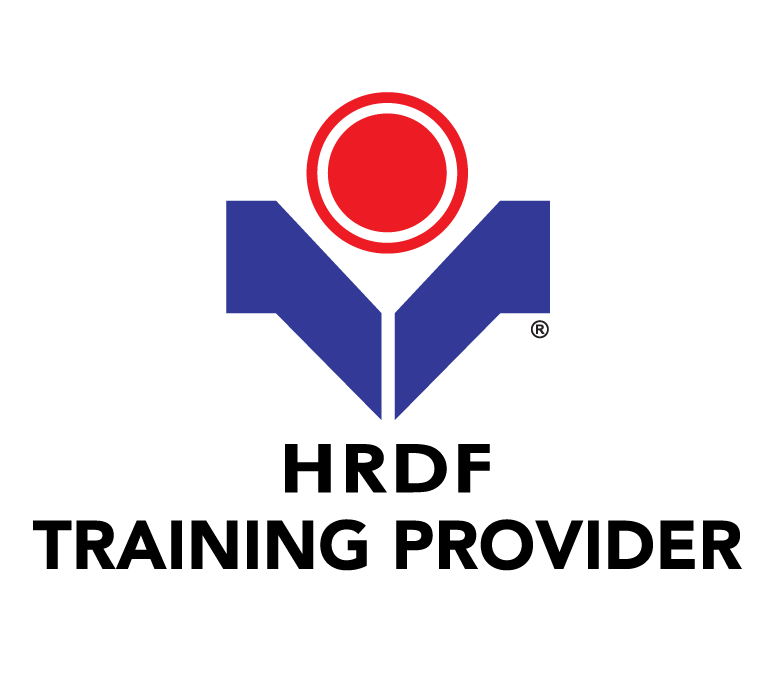# Six sigma Yellow Belt

## About Six sigma Yellow Belt

Six Sigma is a statistical problem-solving methodology. This Six Sigma Yellow Belt course teaches the Six Sigma process of Define-Measure-Analyze-Improve-Control and teaches basic statistical tools in the process. It is an ideal introduction to Six Sigma statistics which will also provide skills to solve non-complex problems using six sigma methodology and statistics.

21 Hours

## Who Should Attend This Course?

• Managers
• Supervisors
• Technicians & Engineers

## What Will You Learn?

• Learn Formal process of solving problems using Six Sigma DMAIC process
• Learn Basic Statistical Methods in Problem Solving
• Learn how to perform a gage R&R and Hypothesis Testing
• Learn basic Statistical Process Controls